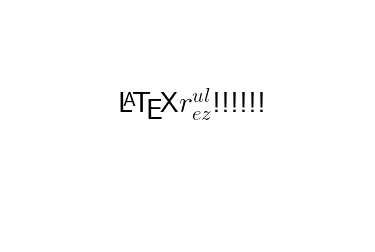Run the cells below to plot with LaTeX in Matplotlib.

Note that you may need to run the bottom cell twice (it'll error the first time due to a caching bug with matplotlib)

In :
import matplotlib.pyplot as plt
plt.rcParams['text.usetex'] = True
plt.ion()

In :
fig, ax = plt.subplots()
ax.text(.5, .5, "\LaTeX $r_{ez}^{ul}$!!!!!!", fontsize=30, horizontalalignment='center')
ax.set_axis_off()In [ ]: